GMAT Math : Arithmetic

Example Questions

Example Question #41 : Dsq: Understanding The Properties Of Integers

A whole number has four digits. True or false: The integer is divisible by 12.

Statement 1: The sum of the digits in the number is 36.

Statement 2: The last digit of the number is 9.

Statement 1 ALONE is sufficient to answer the question, but Statement 2 ALONE is NOT sufficient to answer the question.

EITHER statement ALONE is sufficient to answer the question.

BOTH statements TOGETHER are sufficient to answer the question, but NEITHER statement ALONE is sufficient to answer the question.

Statement 2 ALONE is sufficient to answer the question, but Statement 1 ALONE is NOT sufficient to answer the question.

BOTH statements TOGETHER are insufficient to answer the question.

EITHER statement ALONE is sufficient to answer the question.

Explanation:

Assume Statement 1 alone. If the sum of the digits of a four-digit number is 36, then the average value of each digit is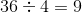; the only possible number that can have this property is 9,999, which is not divisible by 12 since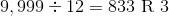.

Now assume Statement 2 alone. For a number to be divisible by 12, it must also be divisible by any factors of 12, including 2. This is only possible if the last digit is even, which it is not. Therefore, the number is not divisible by 12.

Example Question #42 : Dsq: Understanding The Properties Of Integers

A whole number has four digits. True or false: The integer is divisible by 11.

Statement 1: The sum of the digits is 22.

Statement 2: The last two digits are 55.

BOTH statements TOGETHER are sufficient to answer the question, but NEITHER statement ALONE is sufficient to answer the question.

BOTH statements TOGETHER are insufficient to answer the question.

EITHER statement ALONE is sufficient to answer the question.

Statement 1 ALONE is sufficient to answer the question, but Statement 2 ALONE is NOT sufficient to answer the question.

Statement 2 ALONE is sufficient to answer the question, but Statement 1 ALONE is NOT sufficient to answer the question.

BOTH statements TOGETHER are insufficient to answer the question.

Explanation:

Assume both statements to be true. The numbers 6,655 and 7,555 both end in 55 and have digit sum 22. However,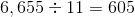and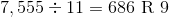Only the former is divisible by 11, so the two statements together do not answer the question.

Example Question #43 : Dsq: Understanding The Properties Of Integers

True or false: Positive integeris prime.

Statement 1: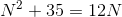Statement 2: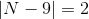Statement 2 ALONE is sufficient to answer the question, but Statement 1 ALONE is NOT sufficient to answer the question.

Statement 1 ALONE is sufficient to answer the question, but Statement 2 ALONE is NOT sufficient to answer the question.

EITHER statement ALONE is sufficient to answer the question.

BOTH statements TOGETHER are sufficient to answer the question, but NEITHER statement ALONE is sufficient to answer the question.

BOTH statements TOGETHER are insufficient to answer the question.

EITHER statement ALONE is sufficient to answer the question.

Explanation:

Assume Statement 1 alone. Then: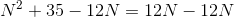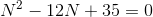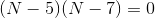Either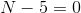, in which case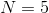, or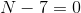, in which case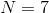Each of 5 and 7 has exactly two factors, 1 and itself, so either wayis prime.

Assume Statement 2 alone. Then:Either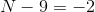or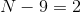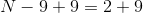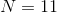Each of 7 and 11 has exactly two factors, 1 and itself, so either wayis prime.

Example Question #44 : Dsq: Understanding The Properties Of Integers

True or false: Positive integeris prime.

Statement 1: The last digit ofis 4.

Statement 2: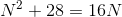.

Statement 2 ALONE is sufficient to answer the question, but Statement 1 ALONE is NOT sufficient to answer the question.

BOTH statements TOGETHER are insufficient to answer the question.

BOTH statements TOGETHER are sufficient to answer the question, but NEITHER statement ALONE is sufficient to answer the question.

Statement 1 ALONE is sufficient to answer the question, but Statement 2 ALONE is NOT sufficient to answer the question.

EITHER statement ALONE is sufficient to answer the question.

Statement 1 ALONE is sufficient to answer the question, but Statement 2 ALONE is NOT sufficient to answer the question.

Explanation:

Assume Statement 1 alone. An integer is divisible by 2 if and only if its last digit is 0, 2, 4, 6, or 8. Therefore, it therefore follows thatis divisible by 2. Since the only prime number divisible by 2 is 2 itself, any integer ending in 4 must not be prime.

Assume Statement 2 alone. Then: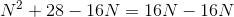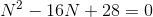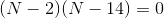Either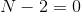, in which case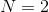- a prime number, or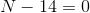, in which case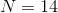- a composite number since it has more than two factors (1, 2, 7, 14).

Therefore, it is not clear whether or notis prime.

Example Question #45 : Dsq: Understanding The Properties Of Integers

A whole number has four digits. True or false: The integer is divisible by 4.

Statement 1: The last digit of the integer is 4.

Statement 2: The sum of the four digits is 20.

BOTH statements TOGETHER are sufficient to answer the question, but NEITHER statement ALONE is sufficient to answer the question.

Statement 1 ALONE is sufficient to answer the question, but Statement 2 ALONE is NOT sufficient to answer the question.

EITHER statement ALONE is sufficient to answer the question.

BOTH statements TOGETHER are insufficient to answer the question.

Statement 2 ALONE is sufficient to answer the question, but Statement 1 ALONE is NOT sufficient to answer the question.

BOTH statements TOGETHER are insufficient to answer the question.

Explanation:

Consider the two four-digit whole numbers 5,564 and 5,474. Each ends in 4, and the sum of the digits in each integer is 20, satisfying the conditions of both statements. However, only the first integer is divisible by 4: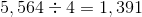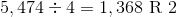The two statements together are therefore insuffcient to answer the question.

Example Question #46 : Dsq: Understanding The Properties Of Integers

True or false: A positive integeris prime.

Statement 1: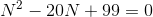Statement 2: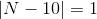Statement 1 ALONE is sufficient to answer the question, but Statement 2 ALONE is NOT sufficient to answer the question.

BOTH statements TOGETHER are insufficient to answer the question.

BOTH statements TOGETHER are sufficient to answer the question, but NEITHER statement ALONE is sufficient to answer the question.

Statement 2 ALONE is sufficient to answer the question, but Statement 1 ALONE is NOT sufficient to answer the question.

EITHER statement ALONE is sufficient to answer the question.

BOTH statements TOGETHER are insufficient to answer the question.

Explanation:

Assume both statements to be true.

From Statement 1: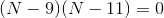Either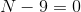, in which case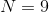, or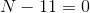, in which case.

From Statement 2:Either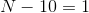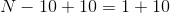or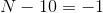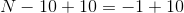Both equations have the same two solutions, 9 and 11, so it is unclear whetheror.

A prime number has exactly two factors, 1 and itself. 9 has 3 as a factor, so 9 is not prime; 11 has only 1 and 11 as factors, so 11 is prime. Since eitheror, it is not clear whetheris prime or not.

Example Question #47 : Dsq: Understanding The Properties Of Integersis a positive integer. True or false:is prime.

Statement 1: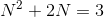Statement 2:is a factor of 3.

Statement 1 ALONE is sufficient to answer the question, but Statement 2 ALONE is NOT sufficient to answer the question.

BOTH statements TOGETHER are insufficient to answer the question.

BOTH statements TOGETHER are sufficient to answer the question, but NEITHER statement ALONE is sufficient to answer the question.

Statement 2 ALONE is sufficient to answer the question, but Statement 1 ALONE is NOT sufficient to answer the question.

EITHER statement ALONE is sufficient to answer the question.

Statement 1 ALONE is sufficient to answer the question, but Statement 2 ALONE is NOT sufficient to answer the question.

Explanation:

Assume Statement 1 alone. Then: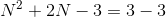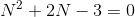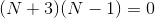Either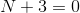, in which case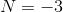, or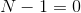, in which case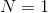.

However, it is given in the problem thatis a positive integer, so, which is not considered to be a prime number, since it does not have exactly two factors.

Assume Statement 2 alone. 3 has only two factors, 1, which is not considered a prime number, and 3, which, having only two factors, is a prime number. Eitheror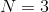, so without further information, it is not clear whetheris prime.

Example Question #48 : Dsq: Understanding The Properties Of Integers

A whole number has four digits. True or false: The integer is divisible by 6.

Statement 1: The last digit in the number is 6.

Statement 2: The sum of the digits in the number is 24.

Statement 2 ALONE is sufficient to answer the question, but Statement 1 ALONE is NOT sufficient to answer the question.

BOTH statements TOGETHER are insufficient to answer the question.

Statement 1 ALONE is sufficient to answer the question, but Statement 2 ALONE is NOT sufficient to answer the question.

EITHER statement ALONE is sufficient to answer the question.

BOTH statements TOGETHER are sufficient to answer the question, but NEITHER statement ALONE is sufficient to answer the question.

BOTH statements TOGETHER are sufficient to answer the question, but NEITHER statement ALONE is sufficient to answer the question.

Explanation:

A whole number is divisible by 6 if and only if it is divisible by all of the factors of 6, which, other than 1 and 6 itself, are 2 and 3. Therefore, it must be established that the number is divisible by both of these numbers. To be divisible by 2, it is necessary and sufficient that the last digit of the number be 0, 2, 4, 6, or 8. To be divisible by 3, it is necessary and sufficient that the sum of the digits be divisible by 3.

Statement 1 alone, therefore, proves that the number is divisible by 2; however, it does not address divisibility by 3. Similarly, Statement 2 alone proves that the number is divisible by 3 (since 24 is as well), but it does not address divisibility by 2. The two statements together, however, address divisibility by 6, since if both are true, it holds that the number is divisible by both 2 and 3, and, consequently, by 6.

Example Question #49 : Dsq: Understanding The Properties Of Integers

A whole number has four digits. True or false: The integer is divisible by 5.

Statement 1: The last digit is 5.

Statement 2: The sum of the digits is 25.

Statement 2 ALONE is sufficient to answer the question, but Statement 1 ALONE is NOT sufficient to answer the question.

EITHER statement ALONE is sufficient to answer the question.

BOTH statements TOGETHER are insufficient to answer the question.

BOTH statements TOGETHER are sufficient to answer the question, but NEITHER statement ALONE is sufficient to answer the question.

Statement 1 ALONE is sufficient to answer the question, but Statement 2 ALONE is NOT sufficient to answer the question.

Statement 1 ALONE is sufficient to answer the question, but Statement 2 ALONE is NOT sufficient to answer the question.

Explanation:

Assume Statement 1 alone. A necessary and sufficient condition for a whole number to be divisible by 5 is that its last digit be either 0 or 5. By Statement 1, the number meets this requirement, so it is divisible by 5. Statement 2, which gives the digit sum, is therefore, irrelevant.

Example Question #1 : Discrete Probability

Data sufficiency question- do not actually solve the question

A bag of marbles consist of a mixture of black and red marbles. What is the probability of choosing a red marble followed by a black marble?

1. The probability of choosing a black marble first is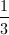.

2. There are 10 black marbles in the bag.

Statements 1 and 2 together are not sufficient, and additional information is necessary to answer the question

Each statement alone is sufficient to answer the question

Statement 1 alone is sufficient, but statement 2 alone is not sufficient to answer the question

Statement 2 alone is sufficient, but statement 1 alone is not sufficient to answer the question

Both statements taken together are sufficient to answer the question, but neither statement alone is sufficient